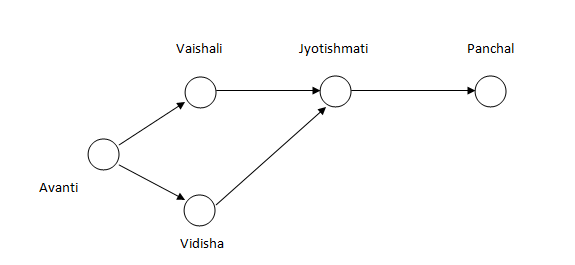### CAT 2001 Question Paper Question 66

Instructions

Directions for the following three questions: Answer the questions based on the pipeline diagram below.

The following sketch shows the pipelines carrying material from one location to another. Each location has a demand for material. The demand at Vaishali is 400, at Jyotishmati is 400, at Panchal is 700, and at Vidisha is 200. Each arrow indicates the direction of material flow through the pipeline. The flow from Vaishali to Jyotishmati is 300. The quantity of material flow is such that the demands at all these locations are exactly met. The capacity of each pipeline is 1,000.Question 66

# The free capacity available at the Avanti-Vaishali pipeline is

Solution

We know that quantity between Vaishali and jyotishmati is 300,

So quantity in avanti-vaishal route should be 400+300=700.

So free capacity is 1000-700=300. Hence option D .